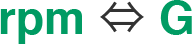# Centrifugal G Force Conversion AppCentrifugal G Force
Conversion AppFind speed (rpm) from centrifugal force (G) and radius (R).

• Centrifugal Force (G)
• G
• mm
• Speed (rpm)

• rpm

• *
Enter centrifugal force (G) and radius (R max.), and click “Convert”.

Find centrifugal force (G) from radius (R) and speed (rpm) .

• Speed (rpm)
• rpm
• mm
• Centrifugal Force (G)

• G

• *
Enter centrifugal force (G) and radius (R max.), and click “Convert”.
• *
The rotor rotating radius is shown on the Rotor Specifications page of the Centrifuge Operation Manual.
• *
The centrifugal force converted by this App is different from the value shown in the centrifuge operation manual.
(Figures are rounded to the nearest whole number in the operation manual.)

Calculation FormulaFixed Angle RotorSwing Out Rotor